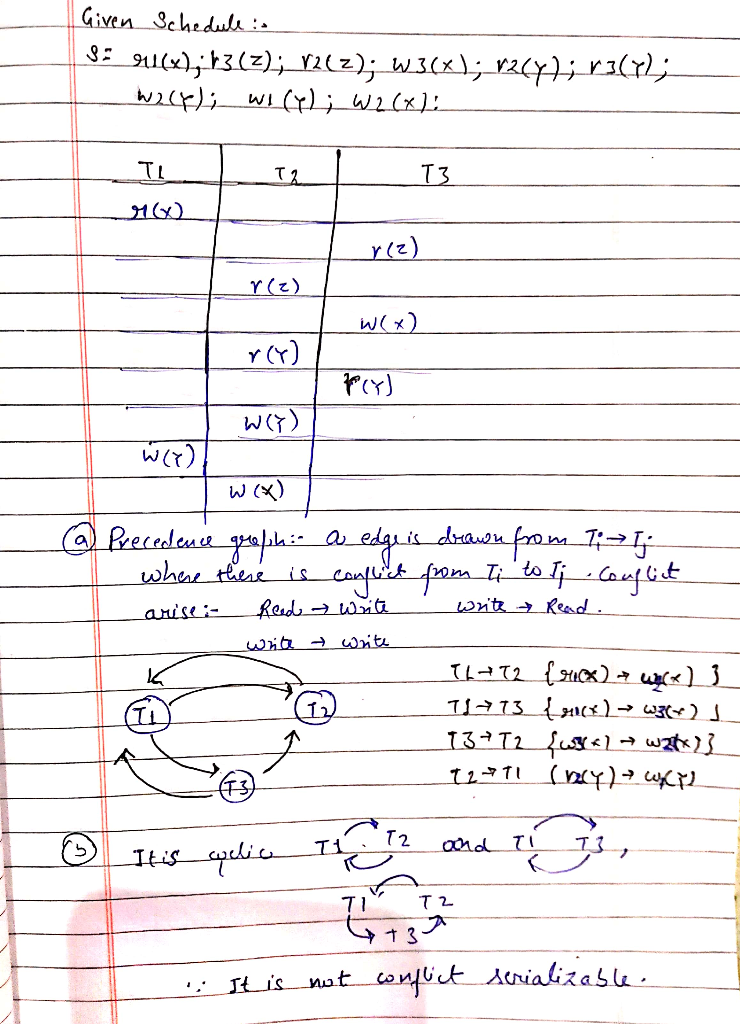# 2. Given the following three transactions T1 = r1(x); w1(y); T2 = r2(z); r2(y); w2(y); w2(x); T3 ...

2. Given the following three transactions

T1 = r1(x); w1(y);
T2 = r2(z); r2(y); w2(y); w2(x);

T3 = r3(z); w3(x); r3(y);

Consider the schedule
S = r1(x); r3(z); r2(z); w3(x); r2(y); r3(y); w2(y); w1(y); w2(x);

a. Draw the precedence graph of schedule S, and label each edge with data item(s).
b. Based on the precedence graph, determine whether S is conflict serializable and justify your answer. If it is serializable, specify all possible equivalent serial schedule(s).##### Add Answer of: 2. Given the following three transactions T1 = r1(x); w1(y); T2 = r2(z); r2(y); w2(y); w2(x); T3 ...
More Homework Help Questions Additional questions in this topic.